Courses

# Reflection and Refraction - EM Waves, Electromagnetic Theory, CSIR-NET Physical Sciences Physics Notes | EduRev

## Physics for IIT JAM, UGC - NET, CSIR NET

Created by: Akhilesh Thakur

## Physics : Reflection and Refraction - EM Waves, Electromagnetic Theory, CSIR-NET Physical Sciences Physics Notes | EduRev

The document Reflection and Refraction - EM Waves, Electromagnetic Theory, CSIR-NET Physical Sciences Physics Notes | EduRev is a part of the Physics Course Physics for IIT JAM, UGC - NET, CSIR NET.
All you need of Physics at this link: Physics

Maxwell's equations can be used to derive the laws of reflection and refraction, which tell us how light waves behave at the boundary between two media with different indices of refraction.

Reflection

Reflection is the abrupt change in the direction of propagation of a wave that strikes the boundary between two different media.  At least some part of the incoming wave remains in the same medium.  Assume the incoming light ray makes an angle θi with the normal of a plane tangent to the boundary.  Then the reflected ray makes an angle θr with this normal and lies in the same plane as the incident ray and the normal.

Law of reflection: θi = θr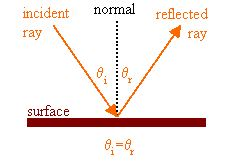Specular reflection occurs at smooth, plane boundaries.  Then the plane tangent to the boundary is the boundary itself.  Reflection at rough, irregular boundaries is diffuse reflection.  The smooth surface of a mirror reflects light specularly, while the rough surface of a wall reflects light diffusely.  The reflectivity or reflectance of a surface material is the fraction of energy of the oncoming wave that is reflected by it.  The reflectivity of a mirror is close to 1.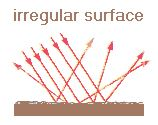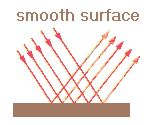Refraction

Refraction is the change in direction of propagation of a wave when the wave passes from one medium into another, and changes its speed.  Light waves are refracted when crossing the boundary from one transparent medium into another because the speed of light is different in different media.  Assume that light waves encounter the plane surface of a piece of glass after traveling initially through air as shown in the figure to the right.

What happens to the waves as they pass into the glass and continue to travel through the glass?  The speed of light in glass or water is less than the speed of light in a vacuum or air.  The speed of light in a given substance is v = c/n, where n is the index of refraction of the substance.  Typical values for the index of refraction of glass are between 1.5 and 1.6, so the speed of light in glass is approximately two-thirds the speed of light in air.  The distance between wave fronts will therefore be shorter in the glass than in air, since the waves travel a smaller distance per period T.

If f is the frequency of the wave and T = 1/f is the period, i.e. the time interval between successive crests passing a fixed point in space, then λ= v1T = cT/n1 and λ= v2T = cT/n2, or λ1= n2/n1.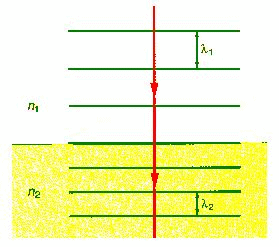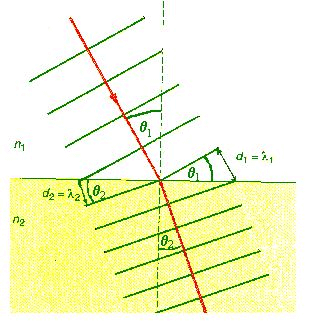Now consider wave fronts and their corresponding light rays approaching the surface at an angle.

We can see that the rays will bend as the wave passes from air to glass.  The bending occurs because the wave fronts do not travel as far in one cycle in the glass as they do in air.  As the diagram shows, the wave front halfway into the glass travels a smaller distance in glass than it does in air, causing it to bend in the middle.  Thus, the ray, which is perpendicular to the wave front, also bends.  The situation is like a marching band marching onto a muddy field at an angle to the edge of the field.  The rows bend as the speed of the marchers is reduced by the mud.  The amount of bending depends on the angle of incidence and on the indices of refraction of glass and air, which determine the change in speed.  From the figure we can see that λ12 = sinθ1/sinθ2.  But λ1= n2/n1. Therefore n2/n= sinθ1/sinθ2, or n1sinθ= n2sinθ2.

This is Snell's law, or the law of refraction.

nisinθ= ntsinθt.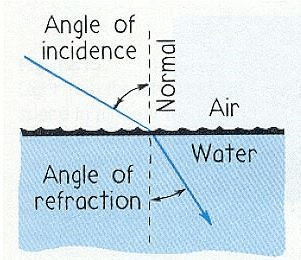When light passes from one transparent medium to another, the rays are bent toward the surface normal if the speed of light is smaller in the second medium than in the first.  The rays are bent away from this normal if the speed of light in the second medium is greater than in the first.  The picture on the right shows a light wave incident on a slab of glass.

One part of the wave is reflected, and another part is refracted as it passes into the glass. The rays are bent towards the normal. At the second interface from glass into air the light passing into the air is refracted again. The rays are now bent away from the normal.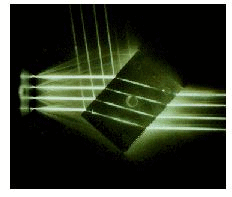Reflectance and transmittance

At a boundary between two transparent media, light is partially reflected and partially refracted.  The ratio of the reflected intensity to the incident intensity is called the reflectance R and the ratio of the transmitted intensity to the incident intensity is called the transmittance T.  Energy conservation requires that R + T = 1 (if there is no absorption).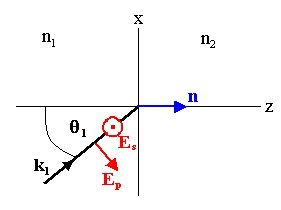R and T depend on the indices of refraction of the two media n1 and n2, the angle of incidence θ1, and the polarization of the incident light.  We distinguish between p-polarization and s-polarization.  Let the plane of incidence contain the normal to the boundary and the incident wave vector k1.  The electric field vector E1 is perpendicular to k1.  If we choose our coordinate system as shown on the right, then plane of incidence is the xz-plane and E1 may be written as E1EpEs.  Ep lies in the xz-plane and Es is perpendicular to the xz-plane, i.e. it points in the ±y-direction.  The electric field of the incident light is a linear superposition of p- and s-polarized fields.

For p-polarized light we have R = |r12p|2, where r12p is the Fresnel reflection coefficient for p-polarization.  We have

r12p = tan(θ- θ2)/tan(θ+ θ2).

For s-polarized light we have R = |r12s|2, where r12s is the Fresnel reflection coefficient for s-polarization.  We have

r12s = sin(θ- θ2)/sin(θ+ θ2).

For a graph of the reflectance R for s- and p-polarized light as a function of n1, n2, and θ1, download this Excel spreadsheet.

If θ+ θ2 = π/2,then tan(θ+ θ2) = infinite and r12p = 0.  If light is reflected, it will have s-polarization.  The incident angle at which this happens is called the Brewster angle θB.   We then have

n1sinθ= n2sin((π/2) - θB) = n2cosθB.

tanθB = n2/n1.

Polarized light can thus be obtained via reflection.

159 docs

,

,

,

,

,

,

,

,

,

,

,

,

,

,

,

,

,

,

,

,

,

,

,

,

,

,

,

;